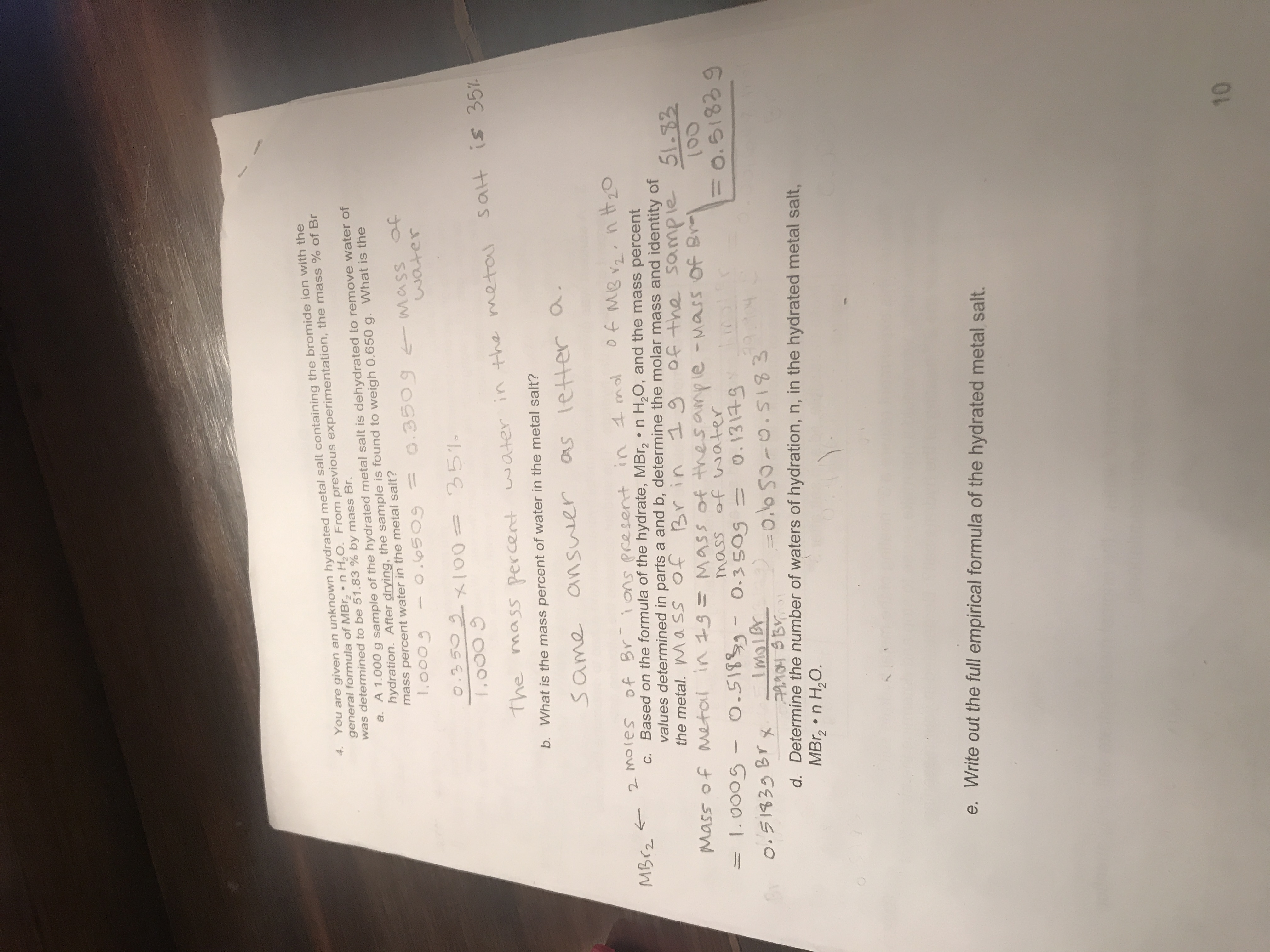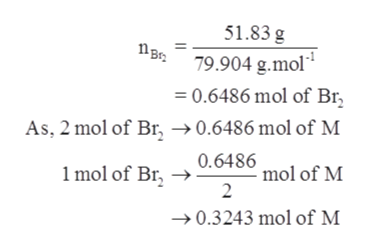# 4. You are given an unknown hydrated metal salt containing the bromide ion with thegeneral formula of MBr n H2O. From previous experimentation, the mass % of Brwas determined to be 51.83 % by mass Br.a. A 1.000 g sample of the hydrated metal salt is dehydrated to remove water ofhydration. After drying, the sample is found to weigh 0.650 g. What is themass percent water in the metal salt?1,0005O.350 9 4 MasswaterO.65093503100= 351sat is 357The masS percertwater in the mQtab. What is the mass percent of water in the metal salt?os letter aSame answer2 Molesc. Based on the formula of the hydrate, MBr2 n H2O, and the mass percentvalues determined in parts a and b, determine the molar mass and identity ofthe metal. Wa ss of Br inof Br ins Present in molMBr219 0f the51.32sampleMass of metol in 1S=Mass of thesa le -Mass Of BrMass of waterO-3505O-51889-ImolBr.0005F0.518390.1314-9O o.51839 Brx=0.0 50-0.S183d. Determine the number of waters of hydration, n, in the hydrated metal salt,MBr2 n H2O.Write out the full empirical formula of the hydrated metal salt.10

Question
409 viewshelp_outlineImage Transcriptionclose4. You are given an unknown hydrated metal salt containing the bromide ion with the general formula of MBr n H2O. From previous experimentation, the mass % of Br was determined to be 51.83 % by mass Br. a. A 1.000 g sample of the hydrated metal salt is dehydrated to remove water of hydration. After drying, the sample is found to weigh 0.650 g. What is the mass percent water in the metal salt? 1,0005 O.350 9 4 Mass water O.6509 3503100= 351 sat is 357 The masS percert water in the mQta b. What is the mass percent of water in the metal salt? os letter a Same answer 2 Moles c. Based on the formula of the hydrate, MBr2 n H2O, and the mass percent values determined in parts a and b, determine the molar mass and identity of the metal. Wa ss of Br in of Br ins Present in mol MBr2 19 0f the 51.32 sample Mass of metol in 1S=Mass of thesa le -Mass Of Br Mass of water O-3505 O-51889- ImolBr .0005 F0.51839 0.1314-9 O o.51839 Brx =0.0 50-0.S183 d. Determine the number of waters of hydration, n, in the hydrated metal salt, MBr2 n H2O. Write out the full empirical formula of the hydrated metal salt. 10 fullscreen
check_circle

Step 1

Given information:

Mass percent of bromine in the compound = 51.83 %

Step 2

As the mass percent of water was calculated 35 % in the question sample thus, the mass of metal will be calculated as follows:

Step 3

c. To calculate the molar mass of metal the follow...help_outlineImage Transcriptionclose51.83 g B79.904 g.mol* = 0.6486 mol of Br As, 2 mol of Br -»0.6486 mol of M 1mol of Br, 0.6480 mol of M 2 0.3243 mol of M fullscreen

### Want to see the full answer?

See Solution

#### Want to see this answer and more?

Solutions are written by subject experts who are available 24/7. Questions are typically answered within 1 hour.*

See Solution
*Response times may vary by subject and question.
Tagged in

### General Chemistry